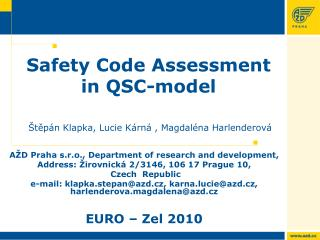Download PresentationSafety Code Assessment in QSC-model Štěpán Klapka, Lucie Kárná , Magdaléna Harlenderová

# Safety Code Assessment in QSC-model Štěpán Klapka, Lucie Kárná , Magdaléna Harlenderová - PowerPoint PPT PresentationDownload Presentation## Safety Code Assessment in QSC-model Štěpán Klapka, Lucie Kárná , Magdaléna Harlenderová

- - - - - - - - - - - - - - - - - - - - - - - - - - - E N D - - - - - - - - - - - - - - - - - - - - - - - - - - -
##### Presentation Transcript

1. Safety Code Assessment in QSC-modelŠtěpánKlapka, Lucie Kárná , Magdaléna Harlenderová AŽD Praha s.r.o., Department of research and development, Address: Žirovnická 2/3146, 106 17 Prague 10, Czech Republic e-mail: klapka.stepan@azd.cz, karna.lucie@azd.cz, harlenderova.magdalena@azd.cz EURO – Zel 2010

2. Contents • IntroductionNew version - FprEN 50159 • Non-binary linear codes • The probability of undetected errors • Binary Symmetrical Channel (BSC) • q-nary Symmetrical Channel (QSC) • Good and proper codes • Reed-Solomon code example • Conclusion

3. New version - FprEN 50159 • Mergingtwo parts of the former standard (for open and close transmission systems) • Modifications of the standard • Common terminology • Classification of transmission systemsthree categories of transmission systems are defined • More precise requirements for safety codesstandard recommends BSC and QSC model

4. Non-binary linear codes • T: finite field with q elements (code alphabet). • q-nary linear (n,k)-code: k-dimensional linear subspace C of the space Tn • codewords: elements of C. • Usually T=GF(2m). In this case every symbol from GF(2m) can be substituted by its linear expansion and given 2m-nary (n,k)-code can be analysed as a binary (nm,km)-code. • most popular non-binary codes: Reed-Solomon (RS) codes

5. Undetected Errors • Structure of undetected errors • all undetected errors of a linear (n,k)-code = all nonzero codewords of the code • Probability of an undetected error Ai: number of codewords with exactly i nonzero symbols Pi: probability that there are exactly i wrong symbols in the word.

6. Binary Symmetrical Channel (BSC) • BSC: model based on the bit (binary symbol) transmission • The probability pe that the bit changes its value during the transmission (bit error rate) is the same for both possibilities (0→1, 1→0).

7. Q-nary Symmetrical Channel (QSC) QSC: model based on the q- symbols transmission e: probability that a symbol changes value during the transmission

8. Undetected Errors Probability (BSC/QSC) BSC model – Pud(1/2) QSC model – Pud((q-1)/q)

9. Good and proper codes • ”good” q-nary linear (n,k)-code:inequality Pud(e) < qk-n is valid for every e [0,(q-1)/q]. • ”proper” q-nary linear (n,k)-code:function Pud(e) is monotone fore [0,(q-1)/q]. • Unfortunately goodness and properness are relatively rare conditions. • example: perfect codes, MDS codes

10. Example Objective: to show how different results is possible to get in QSC and BSC models Example: RS code on GF(256) with generator polynomial: g(x)=x4+54x3+143x2+x+214. RS codes are Maximum Distance Separable codes (MDS)=> they are ”proper” in the QSC model.

11. RS code x4+54x3+143x2+x+214 Codewords with binary weight 7 w_1=(32, 35, 4, 32, 1) w_2=(64, 70, 8, 64, 2) w_3=(128, 140, 16, 128, 4) w_1=(00100000 00100011 00000100 00100000 00000001) w_2=(01000000 01000110 00001000 01000000 00000010) w_3=(10000000 10001100 00010000 10000000 00000100) 12

12. RS code x4+54x3+143x2+x+214 Binary weight spectrum 14

13. RS code x4+54x3+143x2+x+214 Q-nary weight 5 17

14. RS code x4+54x3+143x2+x+214 SUMMARY QSC/BSC QSC model – proper codefor codeword length255 BSC model – not good code for all codeword length 18

15. Conclusions • The analysis of the probability Pud in the BSC model cannot be replaced by the analysis in the QSC model. • The QSC model could be a suitable alternative when a character oriented transmission is used. • The QSC and BSC models of a communication channel are rather abstract criteria of the linear code structure than the mathematical models, which could describe a real transmission system. • For the code over the GF(2m), it is possible to use the both models. • Without an a priori information about the transmission channel there is no reason to prefer any one from these models.

16. Safety Code Assessment in QSC-model Thank You for Your attention! 20